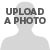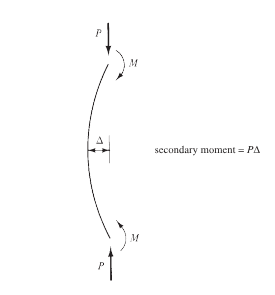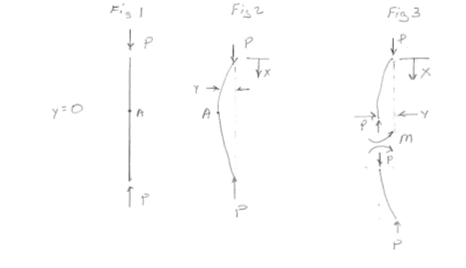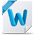## Axially loaded slender column VS Short column#### Kuranage Akila Nadishan Perera04-09-2019 10:00 AM#### Neil Kazen04-10-2019 10:24 AM#### Connor Dick04-10-2019 10:32 AM• #### 1.  Axially loaded slender column VS Short column

Posted 04-09-2019 10:00 AM
Edited by Tirza Austin 04-09-2019 09:59 AM
Why is bucking an important failure mode axially loaded slender column than axially loaded short column?

------------------------------
Ja-Ela
0777487220
------------------------------

• #### 2.  RE: Axially loaded slender column VS Short column

Posted 04-10-2019 10:24 AM
Edited by Tirza Austin 04-10-2019 10:23 AM
Check Euler's equation for column buckling.

------------------------------
Neil Kazen, M.Eng., M.Sc., P.Eng.
FASCE, FCPCI, FEC,
Retired Structural Engineering Manager, Transportation Division, SNC-Lavalin
------------------------------

• #### 3.  RE: Axially loaded slender column VS Short column

Posted 04-10-2019 10:32 AM
Edited by Tirza Austin 04-10-2019 10:31 AM
The longer the unbraced length of a column compared to to its radius of gyration dictates whether a column is considered slender. Once a column is considered slender, its lateral deflection due to axial loads must be considered. These secondary moment effects magnify on itself the more the column deflects. With short columns, these secondary moment effects do not control, therefore is not necessary to be considered as a failure mode. See illustration below:------------------------------
Connor Dick EIT,A.M.ASCE
Jersey City NJ
crd5152@...
------------------------------

• #### 4.  RE: Axially loaded slender column VS Short column

Posted 04-11-2019 04:03 PM
Edited by Tirza Austin 04-11-2019 04:05 PM
​Beam buckling is bending is one way to start off the conversation about buckling. Figure 1 shows a column that is axially loaded in compression. A line through the applied load line of action at one end passes through the line of action of the load at the other end of the column coincides with the column axis. Therefore no bending moment is applied to the column; y=0 over the length of the column. Figure 2 shows a column loaded in compression in which the column has assumed a buckled shape. The buckled shape as shown in Figure 3 moves the columns axis away from the line through which the axial loads act. The displacement of the column axis from the line of action of the loads results in a moment being applied to the column equal to Py. The column develops an internal moment to resist the applied moment Py. The column is subjected to a bending moment and the curvature equation that relates bending moment to curvature can be applied.

d2y/dx2 = M/EI = -Py/EI = (P/EI)y

The solution is:

y = C1sin(P/EI)1/2x + C2cos(P/EI)1/2x

The first Boundary Condition is at x = 0, y = 0:

0 = C1sin(P/EI)1/2(0) + C2cos(P/EI)(0)

C2 = 0

y = C1sin(P/EI)1/2x

The second Boundary Condition is that at x = L, y =0:

0 = C1sin(P/EI)1/2L

The expression is true if either C1 is 0 or sin(P/EI)1/2L = 0. C1 is the amplitude of the sine function. If it is zero, then y is zero and no buckling takes place; the column fails in compression. Therefore, this case is not of interest when discussing buckling. The other term that can be zero is:

sin(P/EI)1/2L = 0

The sine function is zero anytime the (P/EI)1/2L is equal to nπ, where n is an integer. The smallest value of n other than 0 is 1 so:

(P/EI)1/2L = π

The below equation is the standard buckling equation that defines when the column will assume the buckled shape.

P = π2EI/L2 = (π2/L)(EI/L)

Substituting P from above into the deflection equation after incorporating the first B.C. for the column the equation becomes:

y = C1sin(πx/L)

C1 is the maximum deflection which corresponds to the maximum moment in the column (PC1). However, C1 can not be solved for directly due to the way the curvature equation was reduced. It is assumed that dy/dx is small thereby (dy/dx)2 is negligible; equal to 0.

1/ρ = (d2y/dx2)/[1+(dy/dx)2]3/2

1/ρ = d2y/dx2

The maximum moment due to the applied compressive load after the column assumes the buckled shape is PC1. Since the buckled shape is in the shape of a sine wave, the maximum moment is at L/2. Once the column has assumed the buckled shape, it is unstable. Any disturbance may lead to immediate collapse by the formation of a plastic hinge at L/2 if the material is malleable (forms a plastic hinge) or bending fracture at this location if the material is brittle.

Going back to the equation P = (π2/L)(EI/L). It can be seen that the compressive load that causes the column to assume the buckled shape is a function of the column bending stiffness. As the column length increases, the bending stiffness for a constant EI decreases (EI/L gets smaller). One way to look at this decrease in bending stiffness is to assume that the bending moment associated with the buckled shape is constant for all columns. C1 would tend to increase for a constant moment as the column bending stiffness decreased which would increase the applied moment. The moment may remain constant if P decreased with the decrease in bending stiffness/increase in C1.

Mmax = (EI/L)(C1/L)π2

Note that the decrease in C1 is not linear with column length.

Another factor that can be looked at is the radius of gyration. The radius of gyration is:

r2 =  I/A

It can be related to the axial load that results in the buckled shape by discussing the compressive stress it induces in the column.

P = π2EI/L2

P/A = π2E(I/A)/L2 = π2E/(L/r)2

The bending stiffness is EI/L (neglecting the 4) and the axial stiffness is AE/L. Dividing the bending stiffness by the axial stiffness results in:

Bending stiffness/Axial stiffness = EI/L)/(AE/L) = I/A = r2

The radius of gyration is the bending stiffness of the column relative to the axial stiffness. As the radius of gyration increases, the bending stiffness increases relative to the axial stiffness. It is easier for the column to compress under axial load than to bend laterally (buckle). Likewise as the radius of gyration decreases, the bending stiffness is decreasing relative to the axial stiffness so it is easier for the column to move laterally than it is to compress.

I hope that the material presented added to the discussion and did not confuse the issues. At first this looked like a rather simple question, but upon reflection it is not so simple nor can it be readily solved due to assumptions. You may want to consult a mathematician about the curvature equation.------------------------------
Gary Anderson M.ASCE
South Dakota State Univ
Brookings SD
(605) 688-5659
------------------------------

Attachment(s)